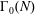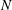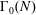Home

# Factorization Tests and Algorithms Arising from Counting Modular Forms and Automorphic Representations

## Abstract

A theorem of Gekeler compares the number of non-isomorphic automorphic representations associated with the space of cusp forms of weight$k$ on$\unicode[STIX]{x0393}_{0}(N)$ to a simpler function of$k$ and$N$ , showing that the two are equal whenever$N$ is squarefree. We prove the converse of this theorem (with one small exception), thus providing a characterization of squarefree integers. We also establish a similar characterization of prime numbers in terms of the number of Hecke newforms of weight$k$ on$\unicode[STIX]{x0393}_{0}(N)$ .

It follows that a hypothetical fast algorithm for computing the number of such automorphic representations for even a single weight$k$ would yield a fast test for whether$N$ is squarefree. We also show how to obtain bounds on the possible square divisors of a number$N$ that has been found not to be squarefree via this test, and we show how to probabilistically obtain the complete factorization of the squarefull part of$N$ from the number of such automorphic representations for two different weights. If in addition we have the number of such Hecke newforms for even a single weight$k$ , then we show how to probabilistically factor$N$ entirely. All of these computations could be performed quickly in practice, given the number(s) of automorphic representations and modular forms as input.

## Footnotes

Hide All

The second author’s work is partially supported by a National Sciences and Engineering Research Council of Canada Discovery Grant.

## References

Hide All
MathJax
MathJax is a JavaScript display engine for mathematics. For more information see http://www.mathjax.org.

# Factorization Tests and Algorithms Arising from Counting Modular Forms and Automorphic Representations

## Metrics

### Full text viewsFull text views reflects the number of PDF downloads, PDFs sent to Google Drive, Dropbox and Kindle and HTML full text views.

Total number of HTML views: 0
Total number of PDF views: 0 *Loading metrics...

### Abstract viewsAbstract views reflect the number of visits to the article landing page.

Total abstract views: 0 *Loading metrics...

* Views captured on Cambridge Core between <date>. This data will be updated every 24 hours.

Usage data cannot currently be displayed